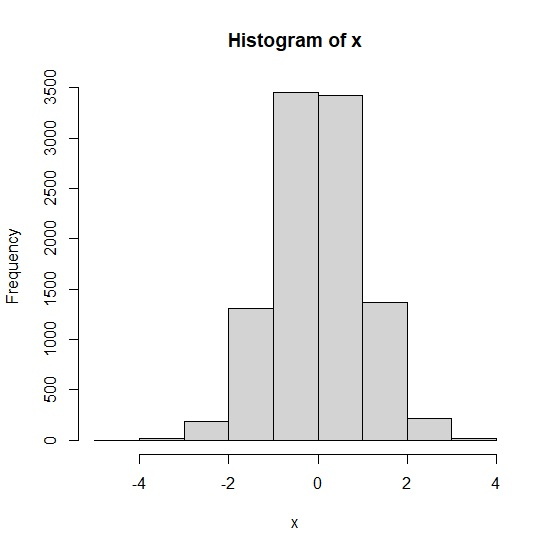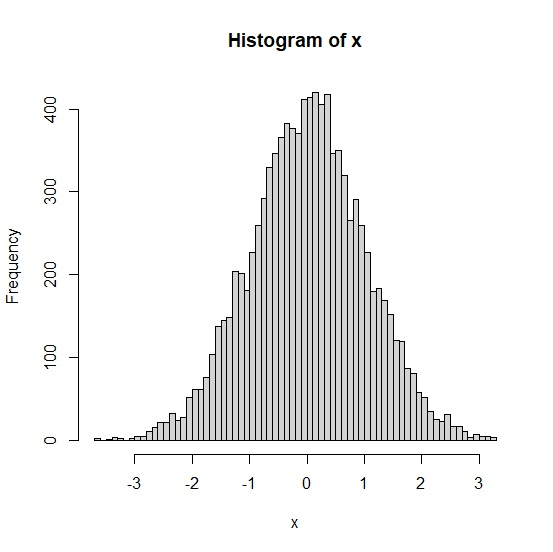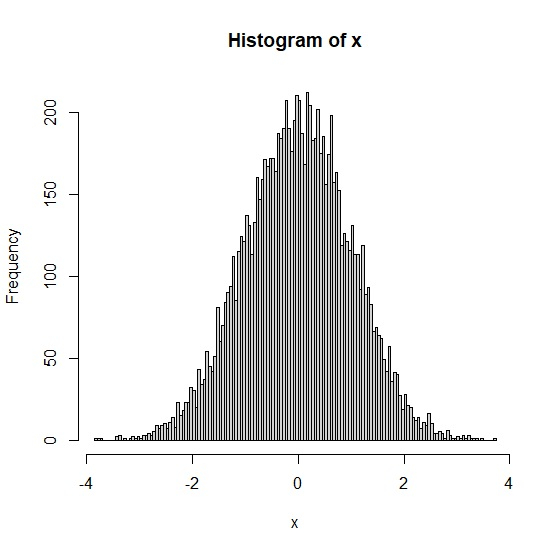# How to define the number of breaks in base R histogram?

To define the number of breaks in base R histogram, we can use breaks argument along with hist function.

## Create the histogram with small number of breaks

Use a vector with normal distribution and the histogram of the same vector with ten breaks −

Live Demo

x<-rnorm(10000)
hist(x,breaks=10)

## Output## Create the histogram with medium number of breaks

Use a vector with normal distribution and the histogram of the same vector with fifty breaks −

Live Demo

x<-rnorm(10000)
hist(x,breaks=50)

## Output## Create the histogram with large number of breaks

Use a vector with normal distribution and the histogram of the same vector with twohundred breaks −

Live Demo

x<-rnorm(10000)
hist(x,breaks=200)

## Output## Create the histogram with medium number of breaks

Use a vector with normal distribution and the histogram of the same vector with fifty breaks −

Live Demo

x<-rnorm(10000)
hist(x,breaks=50)

## Output